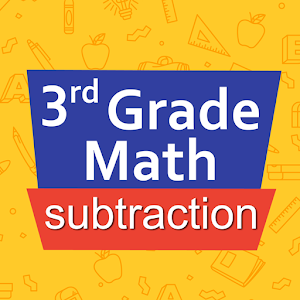Nederlands

We bieden dagelijks GRATIS gelicenseerde Android apps en games aan, die je normaal zou moeten kopen.\$2.99
VERSTREKEN

# Android Giveaway of the Day - Third grade Math - Subtraction

An assistant and training suite for subtraction.
\$2.99 VERSTREKEN
Waarderingscijfer: 0 (0%) 0 (0%)

Deze giveaway is verlopen. Third grade Math - Subtraction is nu beschikbaar op de normale manier.

Intuitive interface powered by handwriting input and a fun and engaging mini game in addition to a regular math trainer mode make our app stand out from the crowd of generic math learning apps.

With Third grade Math - Subtraction you can practice and improve the following math skills:
- Subtraction facts - numbers up to 100
- Subtract two two-digit numbers
- Subtract two three-digit numbers
- Complete the subtraction sentence up to three digits
- Subtract numbers with four or five digits
- Balance subtraction equations up to two digits
- Balance subtraction equations up to three digits

Sergey Malugin

Education

### Versie:

Varies with device

### Grootte:

Varies with device

Everyone

### Compatibiliteit:

Varies with device

## Reactie op Third grade Math - Subtraction

Thank you for voting!